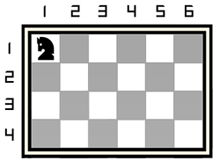시간 제한 메모리 제한 제출 정답 맞은 사람 정답 비율
2 초 256 MB 3 1 1 33.333%

## 문제

There is a chessboard having four rows and $n$ columns. All rows are numbered with the sequential integers from $1$ to $4$, and, similarly, all the columns are numbered with sequential integers from $1$ to $n$. Each square of the chessboard can be described with its coordinates $[r, c]$, where $r$ is its row number and $c$ --- its column number.

Also, there is a chess knight standing at the square $[1, 1]$. The example of $4 \times n$ chessboard with a knight on it is shown below:Knight's target is to make a traversal, that starts and ends at the same square $[1, 1]$. Knight should visit each square (except for the square $[1, 1]$) only once during its traversal.

Chess knight can move from some square to a square that is two squares horizontally and one square vertically, or two squares vertically and one square horizontally. The complete move therefore looks like the letter L.

You are to write a program that will find the maximum number of different visited squares of the knight's traversal and this traversal itself.

## 입력

The only line of input contains the only integer $n$ ($2 \leq n \leq 10^{4}$) --- the number of columns on the chessboard.

## 출력

The first line of output should contain the maximum number of squares $m$ that a knight can visit, traversing every square of the chessboard only once (except for the square $[1, 1]$).

The second line of output should contain the coordinates of the squares to visit in order of the optimum traversal. All the coordinates should be printed as $r_{1} c_{1} r_{2} c_{2} \ldots r_{m} c_{m} r_{1} c_{1}$. Coordinates should be printed with no line breaks and should be separated by the only space.

## 예제 입력 1

6


## 예제 출력 1

22
1 1 2 3 1 5 3 6 2 4 1 2 3 1 4 3 3 5 1 4 2 2 4 1 3 3 4 5 2 6 3 4 4 2 2 1 1 3 2 5 4 4 3 2 1 1